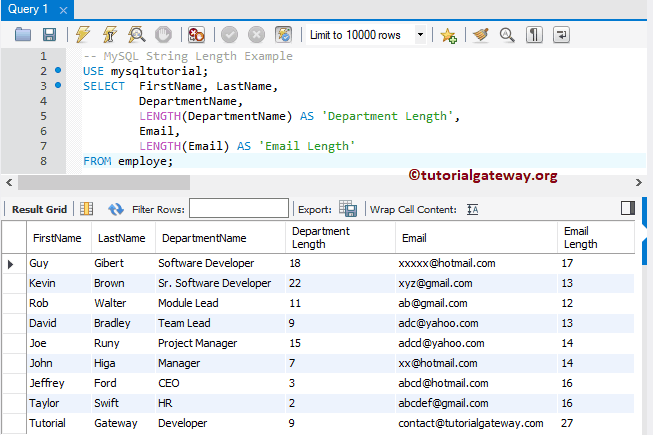# MySQL Length Function

MySQL Length function is one of the String Functions, which is useful to find the length of the user-specified expression (or column value). Or we can simply say, MySQL String Length Function count the number of characters inside an expression, and returns the results. The basic syntax of string Length in MySQL is as shown below:

```SELECT LENGTH (String_Expression)
FROM Source```

For this MySQL String Length Function demonstration, we are going to use the below-shown data

## MySQL Length Example 1

The MySQL string length function counts the number of characters inside a specified expression. The following query shows multiple ways to use this length function.

```-- MySQL String Length Example
SELECT
LENGTH ('Learn MySQL Server') AS `SQL Length`,
LENGTH ('Tutorial Gateway') AS `Website Length`;```

Remember, the Length function count the empty space as well.

## String Length Example 2

The length function also allows you to find the length of the data inside the columns. In this MySQL example, we are going to find the length of two columns Department Name and Email column.

```-- MySQL String Length Example
USE mysqltutorial;
SELECT  FirstName, LastName,
DepartmentName,
LENGTH(DepartmentName) AS 'Department Length',
Email,
LENGTH(Email) AS 'Email Length'
FROM employe;```## MySQL Length Function WHERE Condition

In this String Function example, we show you, How to use length function at the where clause condition. First, we are finding the length of the Department Name and Email columns. Next, we used the length function in where clause to check whether the email length is greater than 15 or not.

```-- MySQL String Length Example
USE mysqltutorial;
SELECT  FirstName, LastName,
DepartmentName,
LENGTH(DepartmentName) AS 'Department Length',
Email,
LENGTH(Email) AS 'Email Length'
FROM employe
WHERE LENGTH(Email) > 15;```

Instead of using the MySQL length in where clause, you might be tempted to use the Alias name in the where clause. But, WHERE Clause does not understand the ALIAS Column name declared in the SELECT Statement.

## MySQL String Length Command prompt Example

In this example, we write the MySQL string length function in Command prompt. Here, we are using the length function along with Where Clause, and Order By Clause.

```-- MySQL String Length Example
USE mysqltutorial;
SELECT  FirstName, LastName,
DepartmentName,
LENGTH(DepartmentName) AS `Department Length`,
Email,
LENGTH(Email) AS `Email Length`
FROM employe
WHERE LENGTH(DepartmentName) > 5
ORDER BY `Department Length` DESC;```